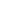#QUA-1012 Probability and Variation is a Course

## QUA-1012 Probability and Variation

Self-paced

### Full course description

Course Description

Probability and variations are the foundation of SPC. The mathematical rules of probability can help to accurately predict the variation in any process.

By the end of this course, you will be able to

• Identify the different types of variation

• Define and explain the six common causes of variation

• Explain the principles of probability

• Calculate the probability of an event

• Construct a histogram

• Explain the concepts of standard deviation

Estimated completion time (hours): 1.8